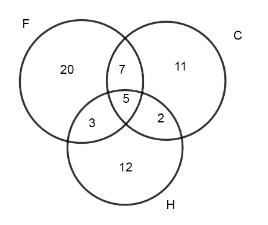Courses
Courses for Kids
Free study material
Free LIVE classes
MoreLIVE
Join Vedantu’s FREE Mastercalss

# In a group of children 35 play football out of which 20 play football only, 22 play hockey; 25 play cricket out of which 11 play cricket only. Out of these 7 play cricket and football but not hockey, 3 play football and hockey but not cricket and 12 play football and cricket both. How many play all the three games?A. 5B. 5C. 12D. 7Verified
333.9k+ views
Hint: At first jot down the given data in the question and then try to make Venn diagram and from that Venn diagram use the formula that $n\left( F\cap C \right)=n\left( F\cap C \right)\left\{ not\text{ hockey} \right\}+n\left( F\cap C\cap H \right)$ to get the answer.

In the question we are told that there are a total 35 children out of which 20 play football only, 22 play hockey and 25 play cricket out of which 11 play cricket only. Out of these 7 play cricket and football but not hockey, 3 play football and hockey but not cricket and 12 play football and cricket both and now from all the data given we will find a number of children who play all these games.
Let football be considered as F, hockey be as H and cricket as C. So according to the data given we can write,
\begin{align} & n\left( F\text{ only} \right)=20 \\ & n\left( H \right)=22 \\ & n\left( C \right)=25 \\ & n\left( C\text{ only} \right)=11 \\ & n\left( C\cap F \right)\left\{ not\text{ hockey} \right\}=7 \\ & n\left( F\cap H \right)\left\{ not\text{ cricket} \right\}=3 \\ & n\left( F\cap C \right)=12 \\ & n\left( F\cap H\cap C \right)=x \\ \end{align}
Let’s represent the above written data in Venn diagram:As we were given that $n\left( F\cap C \right)=12$
Here are ordering to Venn diagram,
$n\left( F\cap C \right)=n\left( F\cap C \right)\left\{ not\text{ hockey} \right\}+n\left( F\cap C\cap H \right)$
So, as we know the values we can substitute them so we get,
$12=7+x\Rightarrow x=5$
So, the correct answer is “Option A”.

Note: While solving these kinds of questions students have questions regarding $n\left( F \right)\text{ and }n\left( F\text{ only} \right)$ as they are both different values. This also goes for $n\left( C \right)\text{ and }n\left( H \right)$ too.
Last updated date: 23rd Sep 2023
Total views: 333.9k
Views today: 5.33k